### IMO Shortlist 1978 problem 12

Kvaliteta:
Avg: 0.0
Težina:
Avg: 0.0
In a triangle$ABC$ we have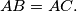$AB = AC.$ A circle which is internally tangent with the circumscribed circle of the triangle is also tangent to the sides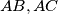$AB, AC$ in the points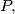$P,$ respectively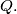$Q.$ Prove that the midpoint of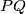$PQ$ is the center of the inscribed circle of the triangle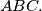$ABC.$
Source: Međunarodna matematička olimpijada, shortlist 1978